3.2Implication Logic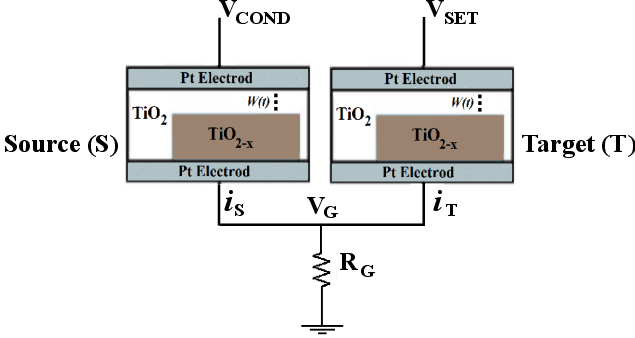Figure 3.2.: Circuit topology of the TiOmemristive implication logic gate.

Recently, it has been shown that a fundamental Boolean logic operation called material implication (IMP) is naturally realized in a simple circuit (Fig. 3.2) combining a conventional resistor and two TiOmemristive switches [75, 76]. This provides stateful logic where non-volatile memory devices are used as the computing elements.

Material implication (IMP) is a fundamental two-input (e.g.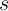and) Boolean logic operation (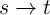), which reads ‘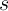implies’ or ‘if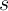, then’, and is equivalent to ‘(NOT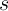) OR’ (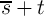) as shown in Table 3.1. The symbols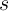andare chosen as they represent the logic states of a source (S) and a target (T) memory element in the stateful logic gate. The operations IMP and NIMP (negated IMP) form a computationally complete logic basis in combination with any operation from the sets C and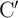, respectively, for which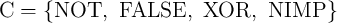and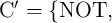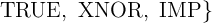and are therefore able to compute arbitrary Boolean functions.

Besides the AND, OR, and NOT operations, the IMP operation has been classified by Whitehead and Russell as one of the four basic logic operations in 1910 . However, by modeling Boolean logic with circuits built with relays and switches, Shannon founded modern digital electronics  only based on AND, OR, and NOT operations due to their straightforward implementation. Since then, the IMP operation has been ignored in digital electronics. Only recently, it was demonstrated that memristive switches intrinsically enable the IMP operation in a crossbar array .

 Table 3.1.: Truth tables of the basic implication operations, IMP and NIMP (negated IMP).

 State s    t st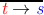1 0    0 1 0 2 0    1 1 1 3 1    0 0 0 4 1    1 1 0

 Table 3.2.: Realized conditional switching behavior is equivalent to the operation IMP or NIMP depending on the definitions for the high and low resistance states (HRS and LRS) as logical ‘0’ and ‘1’.

 Implication operation HRS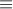0, LRS1 HRS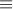1, LRS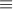0 (conditional switching) t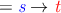t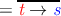State s      t sts  t ts  t t1 HRS   HRS HRS   LRS 0  0 1 1  1 0 2 HRS   LRS HRS   LRS 0  1 1 1  0 0 3 LRS   HRS LRS   HRS 1  0 0 0  1 1 4 LRS   LRS LRS   LRS 1  1 1 0  0 0

Fig. 3.2 shows the circuit topology of the TiOmemristive implication logic gate  combining two TiOmemristors,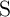and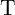, with a conventional resistor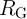. The initial resistance states of the source (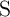) and target (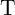) memristors (denoted by the logic variable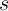and, respectively) are the logic inputs of the gate. The final resistance state of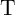after performing the logic operation (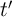) is the logic output of the gate. Performing the logic operation (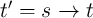) involves simultaneous application of two negative voltage pulses,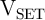and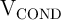, to the non-common terminals of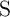and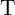.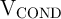is a negative voltage with smaller amplitude than(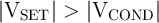). Therefore, the voltage drop on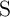is smaller than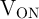(the voltage level required for memristor high-to-low resistance switching) and it remains unchanged after the operation for any input patterns. However, depending on the resistance state of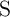, the voltage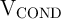changes the voltage level on the common terminal of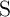and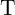(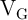) and modulates the voltage drop on the target memristor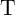. This provides a conditional switching behavior in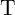, which is shown in Table 3.2. In fact, the negative voltage pulse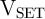enforces a high-to-low resistance switching of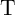only, when both memristors are initially in the high resistance state (State 1). The voltage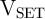has a higher amplitude compared to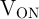as it must compensate the voltage drop on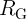.

According to Table 3.2, depending on the logical definitions for the memristor low (LRS) and high (HRS) resistance states, LRS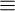logic ‘1’ and HRS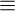logic ‘0’ or vice-versa, the realized conditional switching behavior is corresponding to the IMP or NIMP (negated IMP) operation (Table 3.1). In accordance with the convention of Shannon, if we define HRS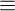1 and LRS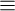0, the logic output of the implication gate corresponds to the NIMP operation as

 {t′ = t NIMP s}≡t → s ≡{t′ = t.s = t AND ss}, (3.1)
where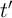is the final state of the variableafter the operation. In combination with the low-to-high resistance switching, which corresponds to the TRUE operation (writing logic ‘1’) according to the above definition, the NIMP operation forms a complete logic basis to compute any Boolean function. Therefore, it enables stateful logic operations by memristive devices used simultaneously as non-volatile memory and logic gates . For instance, stateful universal NOR and NAND operations can be performed in three and five sequential steps as Eq. 3.2 and Eq. 3.3, respectively.
 Step 1 (TRUE) : a = 1 Step 2 (NIMP) : a → b ≡{a′ = a.b = b} Step 3 (NIMP) : a → c ≡{a′ = a.c = b.c = b + c = b NOR c} (3.2) Step 1 (TRUE) : a =1 Step 2 (NIMP) : a → b ≡{a′ = a.b = b} Step 3 (NIMP) : c → a ≡{c′ = c.a = c.b} Step 4 (TRUE) : a =1 Step 5 (NIMP) : a → c ≡{a′ = a.c = c.b = b NAND c} (3.3)
Here,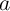(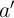) represents the initial (final) logic variable equivalent to the resistance state of a third memristor storing the logic result of intermediary logic steps and the final result of stateful NAND and NOR operations. It should be noted that each logic variable (e.g.) used as an input in Stepis equal to the final logic value (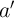) from the previous step (Step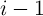) since it has been directly stored in a non-volatile memory element (A).